# Metric Speed (Velocity)

The most common metric units used to measure speed are

• Metres per second (abbreviated as m/s or $\text{ms}^{-1}$) and
• Kilometres per hour (abbreviated as km/h)

Let's have a look at a few examples to get an idea of just how fast these are.

## Metres per Second

Something that travels at a speed of $1 \text{m/s}$ covers a distance of 1 metre in every second.

We usually cover a distance of 4-5,000 metres every hour when we walk. If you walk at 1 metre per second, you are walking a bit more slowly than that as you will only cover 3,600 metres (or 3.6 km) in one hour.

Think of $1 \text{ m/s}$ as a slow walking pace.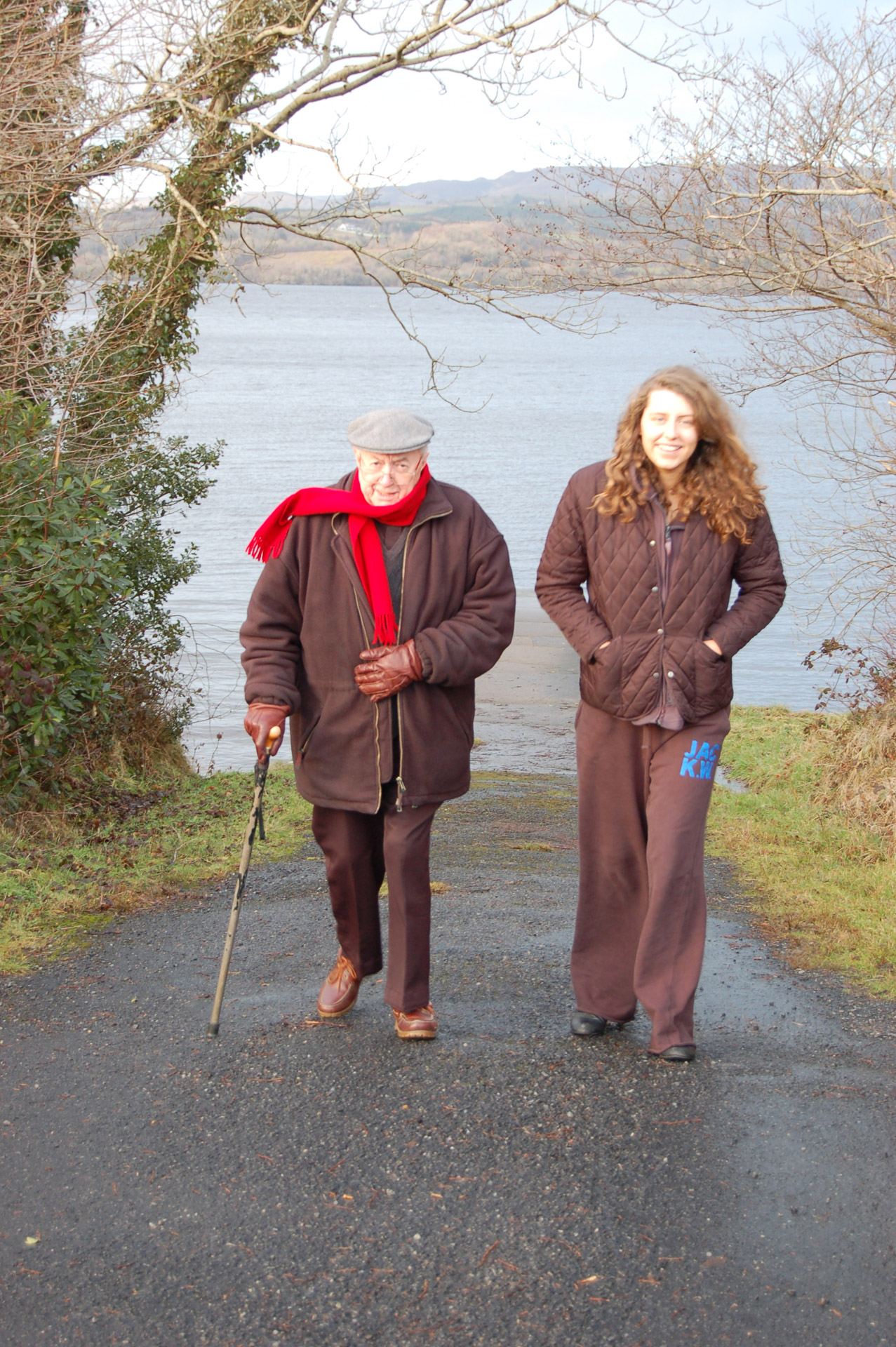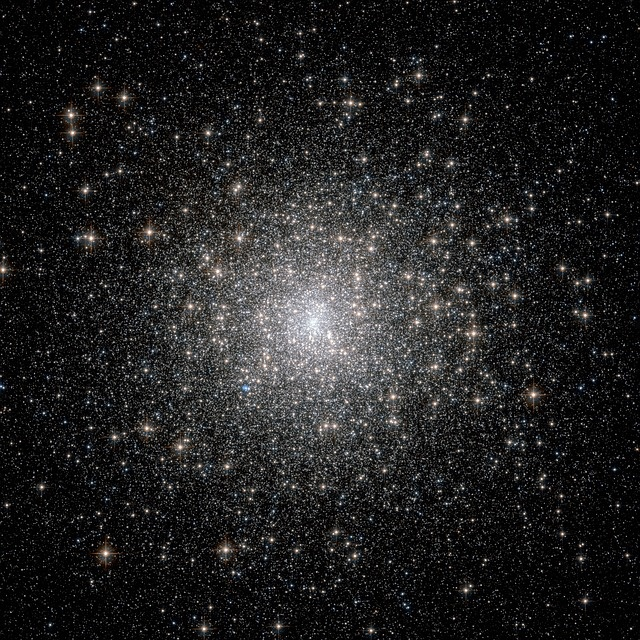Light travels at a speed of $299,792,458 \text{ m/s}$ in a vacuum. According to the theory of relativity, it's impossible to travel any faster than that.

## Kilometres Per Hour

If something is travelling at $1 \text{ km/h}$, it covers a distance of 1 kilometre in every hour. This is a very slow speed.

We often use kilometres per hour to report the speeds of cars and other vehicles.

Let's have a look at a few examples of speeds in kilometres per hour.

The Giant Galapagos Tortoise has a top speed of $1 \text{ km/h}$. He's not going to lumber along to anywhere very quickly, is he?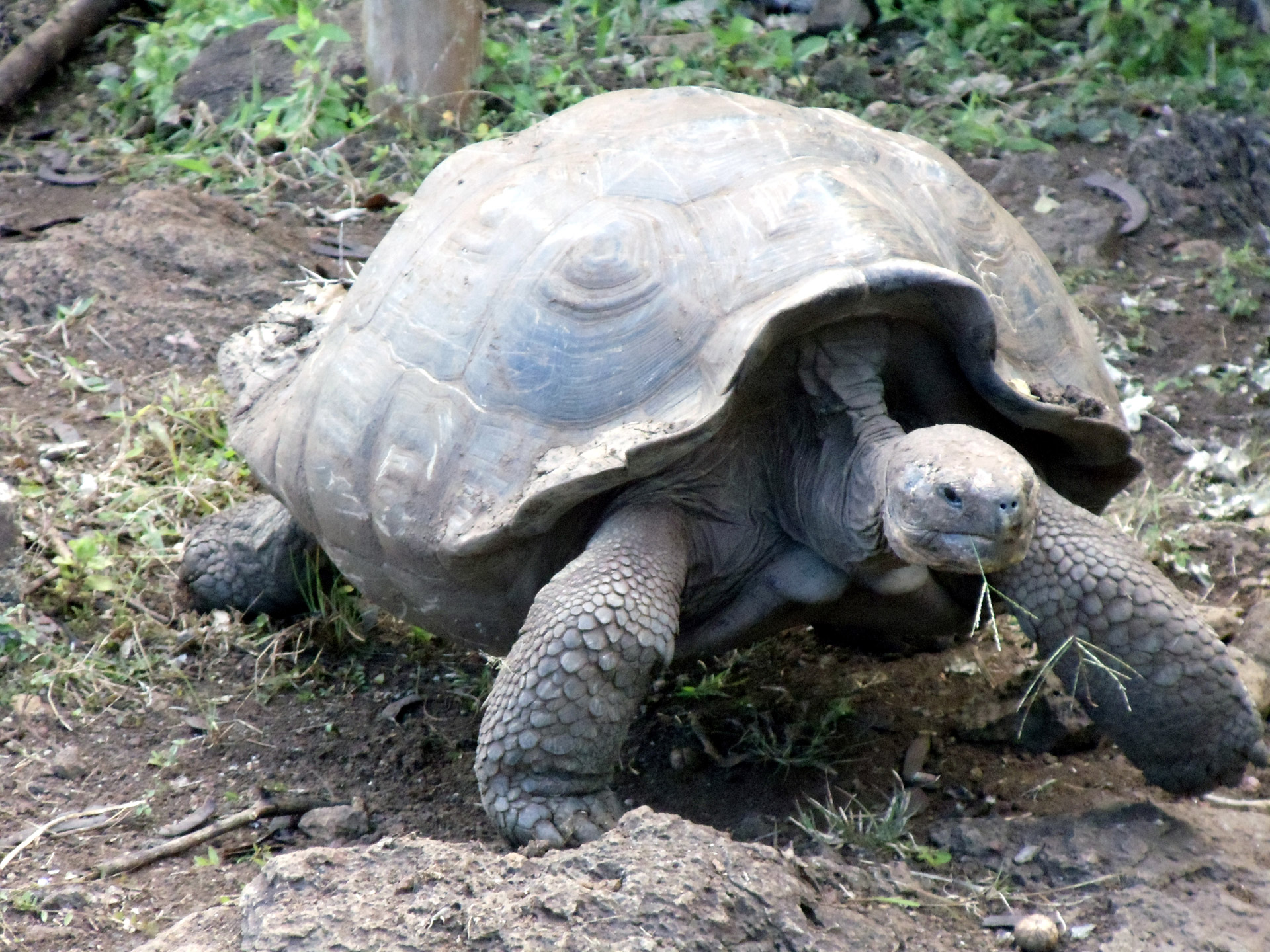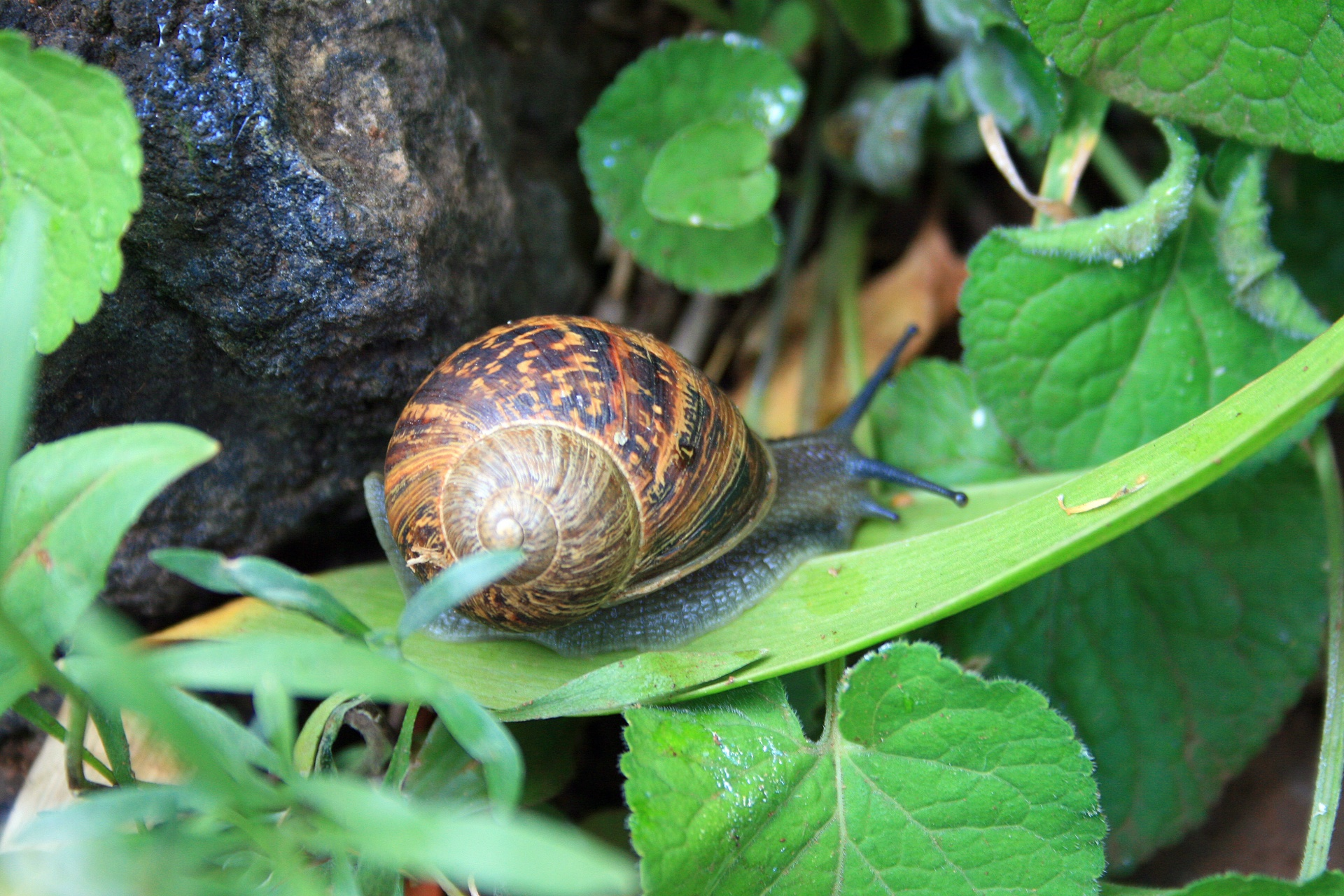Our friendly garden snail is even slower than the giant tortoise. He can only manage a top speed of $0.1 \text{ km/h}$.

$20 \text{ km/h}$ is an easy cycling speed.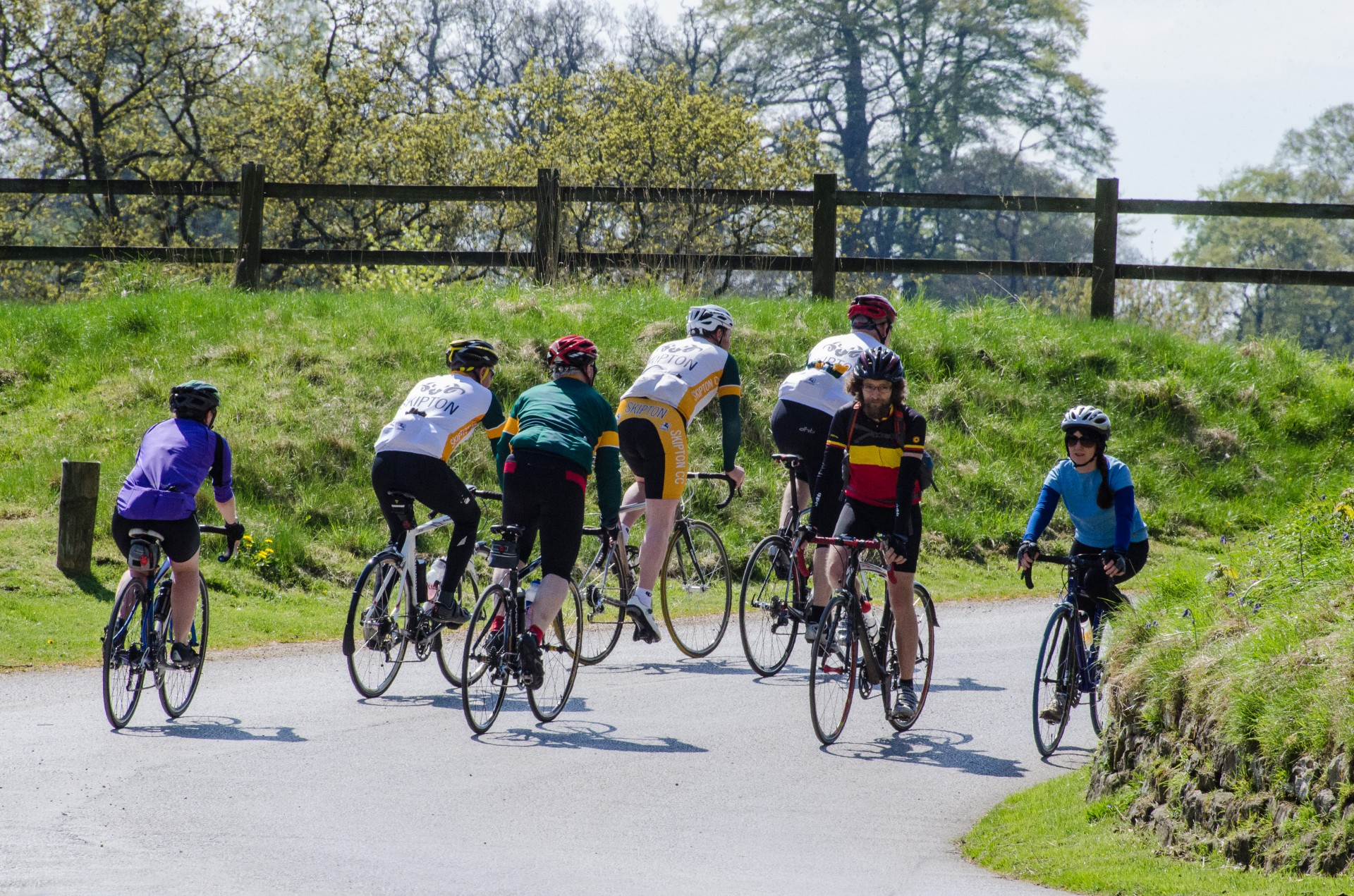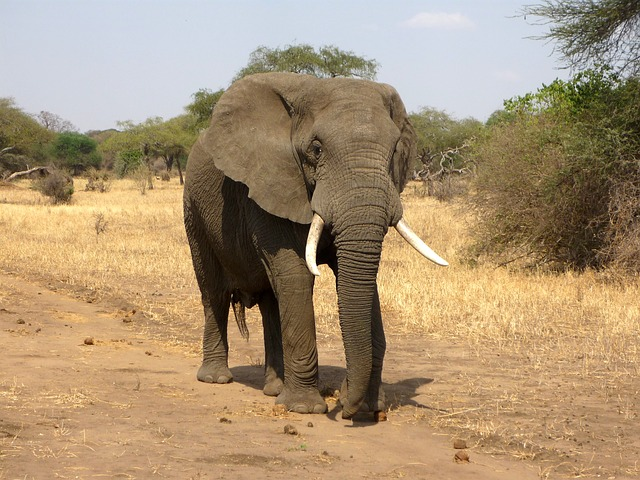$40 \text{ km/h}$ is the speed limit (the maximum speed cars can legally travel at) for school zones in many states. It is also the top speed of an African bush elephant.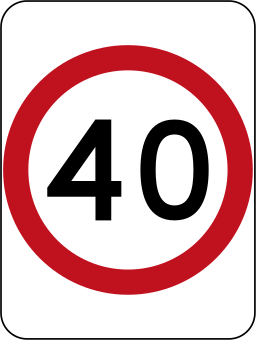$50 \text{ km/h}$ is the maximum speed that cars can travel at in built up areas in many states. It is also the top speed of a large red kangaroo. It is possible to travel at this speed on a bicycle, but you would be going quite fast.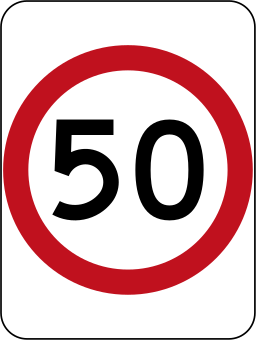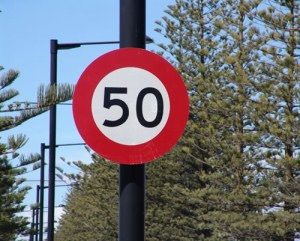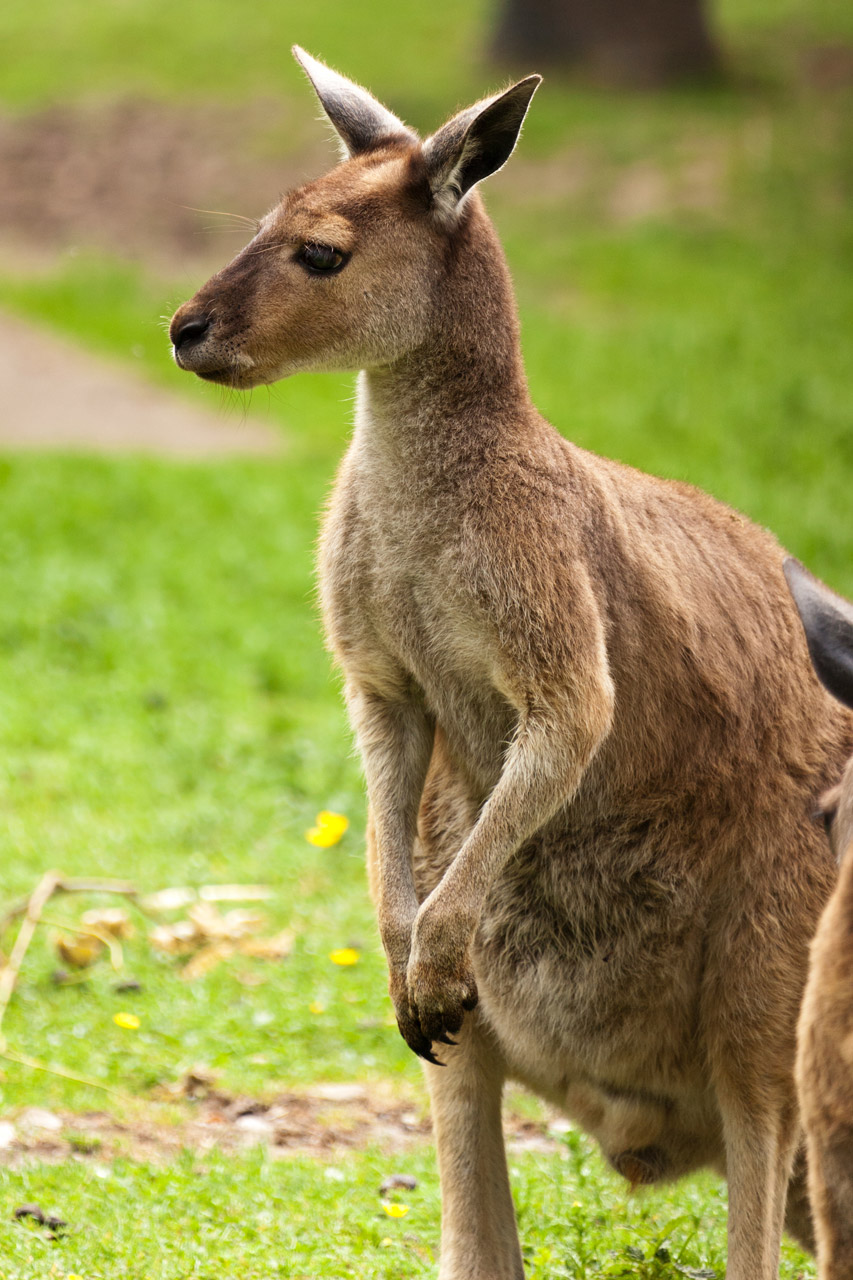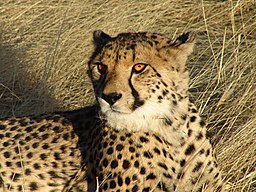A cheetah has a top speed of $100\text{-}120 \text{ km/h}$. The one in the picture is doing $0 \text{ km/h}$. $100 \text{ km/h}$ is the maximum speed at which cars can travel on highways in most states and in New Zealand. It is also about the speed of a goods train.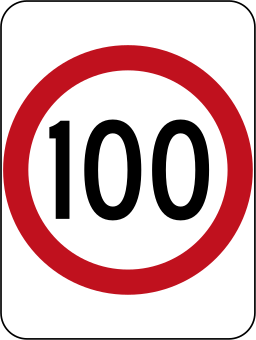The Japanese bullet train (shinkansen) travels at speeds of up to $320 \text{ km/h}$ when it is in service. It has reached much higher speeds in test runs.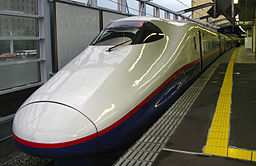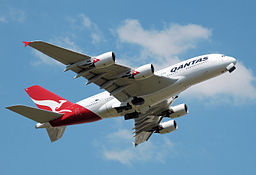The big Airbus A380 plane has a cruising speed of around $1020 \text{ km/h}$.

### Converting Speeds

It isn't too tough to convert speeds from $\text{m/s}$ to $\text{km/h}$. We have the following conversion factors (numbers to multiply by):

• $1 \text{ m/s} = 3.6 \text{ km/h}$
• $1 \text{ km/h}= 0.277777\dots \text{ m/s}$

Let's do a couple of quick conversions as examples:

• A speed of $4 \text{ m/s}$ is equal to a speed of $4 \times 3.6 = 14.4 \text{ km/h}$.
• A speed of $50 \text{ km/h}$ is equal to a speed of $50 \times 0.277777 \dots = 13.888888\dots = 13.89 \text{ m/s} (2 \text{ decimal places})$.

### What's the Difference Between Speed and Velocity?

A velocity is a vector quantity, so it must have a direction as well as a size. So, you can think of velocity as speed that is pointed in some direction.

For example, if someone tells you that they have been cycling at $20 \text{ km/h}$ towards the north, then they are giving you their velocity. If they say that they have been cycling at $30 \text{ km/h}$, then they are giving you their speed.

If someone takes a round trip from their home to the shops and then back again, their average velocity for the trip will be $0 \text{ km/h}$ because they have ended up back where they started from. However, their average speed for the trip is likely to have been much higher. Otherwise, they couldn't have gone anywhere at all!

### Description

• Metric numbers
• Speed
• Conversions

and several other topics

### Audience

Year 10 or higher, some chapters are suitable for Year 8+ students

### Learning Objectives

Learn about measurement and related topics

Author: Subject Coach
You must be logged in as Student to ask a Question.